Courses

# Test: Mass Moment Of Inertia

## 15 Questions MCQ Test Engineering Mechanics - Notes, Videos, MCQs & PPTs | Test: Mass Moment Of Inertia

Description
This mock test of Test: Mass Moment Of Inertia for Mechanical Engineering helps you for every Mechanical Engineering entrance exam. This contains 15 Multiple Choice Questions for Mechanical Engineering Test: Mass Moment Of Inertia (mcq) to study with solutions a complete question bank. The solved questions answers in this Test: Mass Moment Of Inertia quiz give you a good mix of easy questions and tough questions. Mechanical Engineering students definitely take this Test: Mass Moment Of Inertia exercise for a better result in the exam. You can find other Test: Mass Moment Of Inertia extra questions, long questions & short questions for Mechanical Engineering on EduRev as well by searching above.
QUESTION: 1

### The measure of the body’s resistance to angular acceleration is called ___________

Solution:

The measure is known as mass moment of inertia of a body. It’s very useful in the designing of the structures. It also helps in the determination of the strength of the body. Thus a very useful thing in the structural engineering.

QUESTION: 2

### Determine the mass moment of the area of the cylinder given below.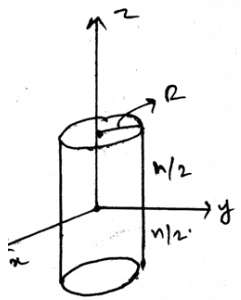Solution:

The measure of moment of inertia of a body is important. It’s very useful in the designing of the structures. It also helps in the determination of the strength of the body. Thus a very useful thing in the structural engineering.

QUESTION: 3

### We define the moment of inertia of a body as________

Solution:

The definition of the of the moment of the inertia of the body is given byThat is the integration of the total mass and the square of the distance of that mass from the axis of rotation. Thus the moment of inertia.

QUESTION: 4

Two of the things of the composite materials are to be known so that their mass moment of inertia can be varied. Which of the following is one of them?

Solution:

The centre of the gravity’s location and the weight of the composite body is needed to be known. This means that if both of the quantities of the composite body is known then the moment of inertia of the body can be altered. Thus both are very important things to be known about the composite bodies.

QUESTION: 5

We define the moment of inertia of a body in terms of volume of the body as______________

Solution:

The definition of the moment of the inertia of the body in terms of volume is given by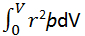That is the integration of the total mass as determined by the product of the volume and density and the square of the distance of that mass from the axis of rotation. Thus the moment of inertia.

QUESTION: 6

We use the distance of the axis and the particles, and in that we apply parallel axis theorem. The distance in the parallel axis theorem is multiplied by_______

Solution:

Parallel axis for any area is used to add the two mutually perpendicular moment of inertias for areas. It gives a moment of inertia perpendicular to the surface of the body. And uses the square of the distance from the axis of rotation multiplied by the area.

QUESTION: 7

One of the use of the centre of mass or centroid is as in the mass moment of inertia is that the net force acts at the ___________ of the loading body.

Solution:

In the moment of inertia calculations we see that the net force acts at the centroid of the loading body. That is if the loading system is in the form of the triangle then the at the distance 2 by 3 of the base the net force of the loading will act. And the load will be half the area of the loading.

QUESTION: 8

If the non-Uniform loading is of the type of parabola then for calculating the mass moment of inertia for areas?

Solution:

The net force will act at the centroid of the parabola. Whether it be a parabola or the cubic curve the centroid is the only point at which the net force act. Force can’t be acted horizontally if the loading is vertical. Hence whatever be the shape of the loading, the centroid is the point of action of net force. Thus the use of centroid.

QUESTION: 9

Determine the mass moment of the area of the cylinder given below.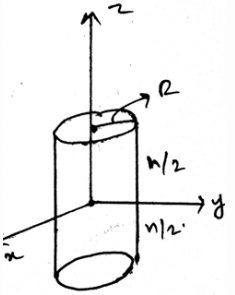Solution:

The measure of moment of inertia of a body is important. It’s very useful in the designing of the structures. It also helps in the determination of the strength of the body. Thus a very useful thing in the structural engineering.

QUESTION: 10

Which of the following is true?

Solution:

The definition of the moment of the inertia of the body in terms of volume is given by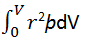That is the integration of the total mass as determined by the product of the volume and density and the square of the distance of that mass from the axis of rotation. Thus the moment of inertia.

QUESTION: 11

The body is sometimes acted by two or three force members and we need to find the mass moment of inertia for the same. The difference between the two and the three force members is:

Solution:

The definition of the two force member only defines that the forces are being acted on the two points on the body. So does is the definition of the three forces members. The points of action of the three forces are three.

QUESTION: 12

If any external force also is applied on the structure and we are determining the mass moment of inertia then what should we consider?

Solution:

The external forces are treated differently. They are not added by the force of the distributed loading. That is the force not only acts at the centroid always. It can be shifted also. Depending on the external forces. Thus the use of centroid or centre of mass.

QUESTION: 13

If any external force also is applied on the distributed loading on which the mass moment of inertia is to be determined on the composite body then?

Solution:

The external forces are treated differently. They are not added by the force of the distributed loading. That is the force not only acts at the centroid always. It can be shifted also. Depending on the external forces. Thus the use of centroid or centre of mass.

QUESTION: 14

Which of the following is true?

Solution:

The definition of the moment of the inertia of the body is given b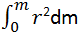That is the integration of the total mass and the square of the distance of that mass from the axis of rotation. Thus the moment of inertia.

QUESTION: 15

We use sometimes the measures to know the direction of mass moment of inertia. It is done by right handed coordinate system. Which is right about it for the composite bodies (consider the mentioned axis to be positive)?

Solution:

As right handed coordinate system means that you are curling your fingers from positive x-axis towards y-axis and the thumb which is projected is pointed to the positive z-axis. Thus visualizing the same and knowing the basic members of axis will not create much problem.